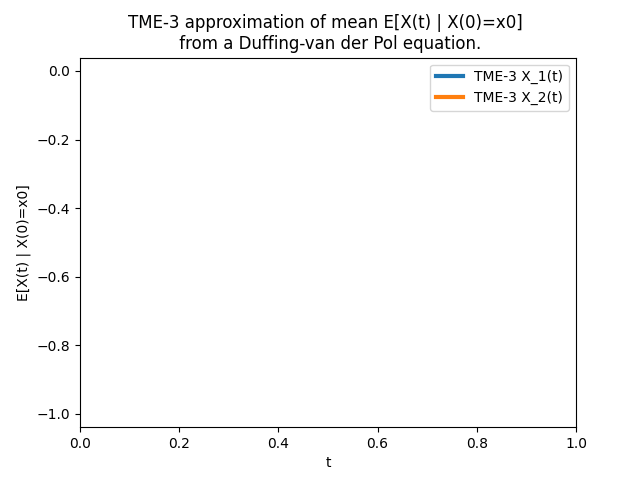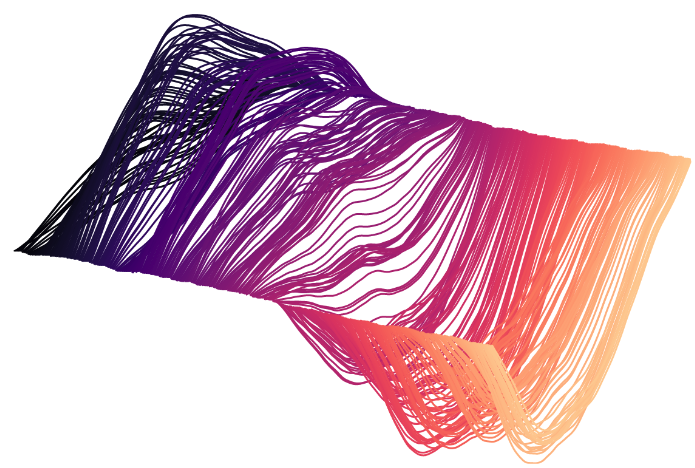### Example article testing element rendering

In this test article, we test if Markdown elements are rendered correctly. First let us see basic inline elements, such as inline code block lambda x: wrapper(x), URL link, Italic, and bold font. Note that the underscore _ is seen as italic decorator hence, please use \_ in math equation to escape this.

Table of contents appears at the place with marker [TOC].

# Section

## Section

### Section

#### Section

The font size of the first level heading seems a bit big. Also it would be good to have automatic heading numbering. The numbering can in principle be done in css but I prefer it generated by backend.

The first paragraph, let's check the line spacing.

Lorem ipsum dolor sit amet, consectetur adipiscing elit. Integer sit amet hendrerit mauris. Quisque sed velit sit amet mi bibendum tempus at et purus. Curabitur eget finibus felis, ac aliquam dui. In in semper urna. Fusce at tellus eu turpis mattis consectetur at ut tortor. Suspendisse est massa, suscipit nec pulvinar vel, efficitur ac lorem. Sed luctus rhoncus magna sit amet cursus. Sed eget blandit lorem, vitae ultricies orci. Donec a nunc malesuada metus ullamcorper vehicula et sed tortor. Suspendisse congue iaculis purus, ut semper sem malesuada non.

Donec ac venenatis lectus. Suspendisse ex orci, sagittis id ullamcorper vel, molestie a diam. Nam semper arcu nulla, id scelerisque dui egestas in. Praesent quis nunc ac lorem auctor luctus. Aenean scelerisque, eros at rhoncus convallis, tellus est molestie diam, nec porttitor lacus augue at massa. Aliquam erat volutpat. Nullam risus sem, commodo nec accumsan non, fermentum quis mi. In suscipit nisi tortor, eu suscipit tortor consequat accumsan. Mauris eget molestie arcu. Nunc venenatis leo ut enim tincidunt, eget suscipit tellus dignissim. Nulla sed nunc nec nisi pharetra auctor. Proin porttitor dolor velit, non lacinia nisl fringilla sed.

Okay well, they look fine.

Next we take a look at displayed code block. This is parsed by Pygments under the default theme. The background colour is the same as in Github.

# Python scripts
import numpy as np

def func(x):
return x ** 2

a = np.array([1., 2., 3.])
print(f'Value is: {a:.2f}')


Another Rust code block

// Rust code
fn test<T>(a: T) -> T
where
T: Mytype
{
let b = b;        // highlight here
let c = 'hello';  // hightlight here
b + 1
}


and another Matlab code block

% Matlab code
a = [1:1:100];
b = a .^ 2;
plot(a, b)


We can also have numbered code block. For details, see Python Markdown.

 1 2 3 4 5 #!/usr/bin/python # Python codes import matplotlib.pyplot as plt plt.plot([1., 2., 3.]) 

Add a figure (the max-width of image is set to 90% of the article width, and is auto-centred.)#### Second section

Ordered list:

1. Element 1
2. Element 2
3. Element 3

Unordered list:

• Elemement 1
• Element 2

Quote block, not sure if its background colour is necessary.

Aliquam porttitor fringilla urna, ut mollis metus placerat ac. Morbi convallis suscipit suscipit. Ut accumsan neque et elit consequat eleifend. Integer eros nibh, scelerisque at pharetra quis, tincidunt vitae metus. Suspendisse nunc felis, interdum eu euismod non, gravida posuere augue. Duis malesuada orci quis justo porttitor, ac cursus magna gravida. Nam vehicula turpis quis elementum faucibus.

Let's see admonition. Colours are picked from Sphinx website. In principle you could add some icons (e.g., exclamation mark) before these titles, but I do not consider this necessary. If you wish, Google "css ::before icon" for how to so so.

Warning

This is a warning message.

Note

This is a note message.

Similarly, you could also use this admonition env to define, for example, theorem and lemma, though using quote block could also do the job.

Lemma 1 (Kolmogorov--Chentsov).

Let $X\colon [0, \infty)\to\mathbb{R}$ be a stochastic process. Suppose that for every $T>0$, there are $\alpha,\beta, C>0$ such that $$\begin{equation} \mathbb{E}\big[ \abs{X(t) - X(s)}^\alpha \big] \leq C \, \abs{t-s}^{1+\beta}, \end{equation}$$ for all $s,t\in[0,T]$. Then there is a version $\widetilde{X}$ of $X$ that is Hölder-continuous of every order $\gamma \in (0, \beta \, / \, \alpha)$ 3.

Proof. Here shows the proof. However, increasing the top margin of the proof environment (or equivalently increase the bottom margin of the second p child) seems to be non-trivial.

Some table.

Syntax Description
Paragraph Text

Here's a sentence with a reference1. The default superscript style is a bit annoying, as I would like to see a number citation. I don't know how to interact with python markdown to add a square bracket around the number, though you could use  in markdown to escape parsing them, but result looks a bit aweful.

Finally let's test some math. Inline math $f(x) = \sin(x) + \mathbb{E} + \mathbf{A} + \mathcal{F}$, $\R^{N\times 2}, \Q, \N, \T$ and displayed math 2

$$\begin{equation} \diff X(t) = A \, X(t) \diff t + B \diff W(t), \tag{5} \label{equ:eq} \end{equation}$$

The equation above looks fine in Chrome and Firefox, but not in Safari 14.1.2 (16611.3.10.1.6). I think this is a Mathjax problem.

I have also pre-defined a few MathJax commands as per the fashion in this .tex. They should be sufficient in many cases. You should edit content/mathjax.html to customise your commands. Note that I am a bit agaist using \left \right to automatically adjust parenthesis height, because these could cause wrong vertal alignment compared to using \big etc. Instead, I prefer to hard-code them, for instance, \mathbb{E}\Big[ #1 \Big]. In the following you could find a few examples.

$$\begin{equation} \begin{split} &\expec{X \cond \FF} \\ &\expecbig{\expp^{\imag X}} \\ &\expecBig{ \big(\expp^{X} - X^2\big)^3 \cond Y}\\ &\cov{X, Y \cond \FF} \\ A\,A^\trans &= A^\trans \, A\\ \norm{A}_2 &\coloneqq \sup_{\norm{u}_2=1} \norm{A \, u}_2\\ &(\jacobian_x\phi)(x)\\ &(\hessian_x\phi)(x)\\ &(A\phi)(x)\\ f_u(v) &= \innerp{u}{v} \\ &\innerpBig{u + z}{\sum^N_{i=1} v_i}\\ \limsup_{N,M\to\infty} X_k &\coloneqq \lim_{N\to\infty} \sup_{M\geq N} X_k \end{split} \end{equation}$$

Now I reference Equation \eqref{equ:eq}.

At last, let me check the support for Chinese. 測試行. 粗體斜體

# Conclusion

Looks like most Markdown elements are rendered properly. There are still some fine tunning to do.

1. This is the footnote/reference. Looks like fine but needs some small adjustments.

2. Another reference.

3. Achim Klenke. Probability theory. Springer, 2014.